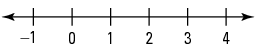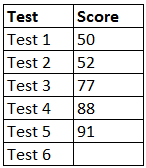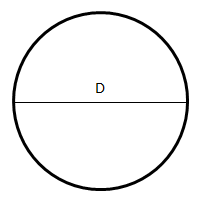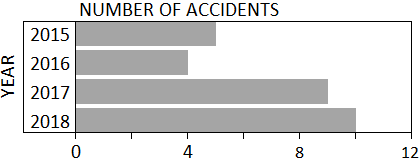# ParaPro Math Practice Test

Try our free ParaPro Math practice test. The math section consists of 30 multiple choice questions covering number sense, basic algebra, geometry, measurement, and data analysis. Our online practice questions include answers and detailed explanations. The use of a calculator is not permitted on the ParaPro Math test.

Congratulations - you have completed .

You scored %%SCORE%% out of %%TOTAL%%.

Your performance has been rated as %%RATING%%

 Question 1

### A farmer has a rectangular field that measures 125 feet by 200 feet. He wants to enclose the field with a fence. What is the total length, in feet, he will need for the job?

 A $350$ B $450$ C $550$ D $650$
Question 1 Explanation:
The correct answer is (D). This question is really asking for the perimeter of the field. Add up all of the sides to find the perimeter:

$(125 \cdot 2) + (200 \cdot 2) = 650$
 Question 2### On the number line above, where is the number $\frac{7}{3}$ located?

 A $\text{Between}$ $0$ $\text{and}$ $1$ B $\text{Between}$ $1$ $\text{and}$ $2$ C $\text{Between}$ $2$ $\text{and}$ $3$ D $\text{Between}$ $3$ $\text{and}$ $4$
Question 2 Explanation:
The correct answer is (C). We know that $\frac{7}{3}$ is equivalent to $2 \frac{1}{3}$ since:

$\require{enclose} \begin{array}{rll} 2 & \hbox{r1} \\[-3pt] 3 \enclose{longdiv}{7}\kern-.2ex \\[-3pt] \underline{-6} \\[-3pt] \,1 \end{array}$

$2 \frac{1}{3}$ falls between $2$ and $3$ on the number line.
 Question 3

### Tavon's flight is 270 minutes long. How many hours does the flight last?

 A $4 \text{ hours}$ B $4 \frac{1}{2} \text{ hours}$ C $5 \text{ hours}$ D $5 \frac{1}{2} \text{ hours}$
Question 3 Explanation:

$\require{cancel} 270 \cancel{\text{minutes}} \cdot \dfrac{1 \text{ hour}}{60 \cancel{\text{minutes}}}$ $= \dfrac{270}{60} \text{hours}$ $= \dfrac{27}{6} \text{hours}$

$\dfrac{27}{6} = \dfrac{9}{2} = 4 \dfrac{1}{2}$
 Question 4

### In a factory there are two separate containers of stress balls. In the first container are 80 balls, and in the second are 90 balls. 40% of the balls in the first container are defective, and 20% in the second container are defective. In total, how many balls in the two containers are defective?

 A $102$ B $50$ C $60$ D $51$
Question 4 Explanation:
The correct answer is (B). Calculate the number of defective balls in each container and then add these two amounts:

$40\% \text{ of } 80$ $= 0.40 \cdot 80 = 32$

$20\% \text{ of } 90$ $= 0.20 \cdot 90 = 18$

$32 + 18 = 50$
 Question 5

### Ayana wants to buy as many bags of mulch as possible with her \$305, and she would like them to be delivered to her house. The cost is \$8.00 per bag and there is a \$21.00 delivery charge. The mulch is only sold in full bags. How many bags can Ayana buy?  A$35$B$36$C$38$D$39$Question 5 Explanation: The correct answer is (A). From Ayana's initial amount of \$305, a flat \$21.00 delivery charge is deducted:$\$305 − \$21.00 = \$284.00$

We can then divide this amount by the cost per bag to find the total number of bags that Ayana can buy:

$\$284.00 ÷ \$8.00$ $= 35.5 \text{ bags}$

However, the question states that the mulch can only be sold in full bags, so we must round our answer down to ensure that Ayana doesn't exceed her budget.
 Question 6

### A family ordered $2$ large pizzas for lunch. They ate $\frac{7}{8}$ of one pizza and $\frac{1}{4}$ of the other pizza. How much pizza was left for dinner?

 A $\dfrac{1}{8}$ B $\dfrac{3}{8}$ C $\dfrac{7}{8}$ D $\dfrac{5}{4}$
Question 6 Explanation:
The correct answer is (C). When dealing with addition and subtraction involving fractions we must remember to always convert everything to a common denominator. If the family consumed $\frac{7}{8}$ of one and $\frac{1}{4}$ or $\frac{2}{8}$ of the other pizza, they consumed a total of $\frac{9}{8} = \frac{11}{8}$. We can now subtract the $\frac{1}{8}$ from the last remaining pizza to find $\frac{7}{8}$.
 Question 7

### Ryan bought a sweatshirt that was priced at \$50. If a sales tax of 6% was added to the price, and Ryan gave the sales clerk three \$20 bills, how much change should he expect to receive?

 A $\$10$B$\$4$ C $\$12$D$\$7$
Question 7 Explanation:
the correct answer is (D). To determine the total amount owed, we need to determine the sales tax:

$6\%$ of $\$50 = .06 \cdot 50 = \$3$

(Or, you might recognize that 6% of \$100 = \$6, so 6% of \$50 must be half of \$6, or \$3.) Total amount owed$= \$50 + \$3 = \$53$

Three $\$20$bills$= 3 \cdot \$20 = \$60\$60 − \$53 = \$7$ change.
 Question 8

### Equation: $x + 17 = 11$ Solution: $x = −6$ An equation and solution are shown above. A student asks for an explanation. Which explanation would be the best for the paraprofessional to provide?

 A $\text{Subtract}$ $17$ $\text{from}$ $\text{both}$ $\text{sides}$ $\text{of}$ $\text{the}$ $\text{equation.}$ B $\text{Subtract}$ $11$ $\text{from}$ $\text{both}$ $\text{sides}$ $\text{of}$ $\text{the}$ $\text{equation.}$ C $\text{Subtract}$ $x$ $\text{from}$ $\text{both}$ $\text{sides}$ $\text{of}$ $\text{the}$ $\text{equation.}$ D $\text{Divide}$ $\text{both}$ $\text{sides}$ $\text{of}$ $\text{the}$ $\text{equation}$ $\text{by}$ $17.$
Question 8 Explanation:
The correct answer is (A). Given $x + 17 = 11$, to solve we want to isolate the variable, $x$. To do this most efficiently, we subtract $17$ from both sides:

$\require{cancel} x + \cancel{17} = 11$
$~~~\require{cancel} − \cancel{17} −17$

$x = −6$
 Question 9

### Tyler struggled with his math class early in the year, but he has been working hard to improve his scores. There is one test left, and he is hoping that his final average test score will be 75. What score will Tyler need to get on Test 6 to finish the year with an average score of 75?A $75$ B $85$ C $92$ D $98$
Question 9 Explanation:
The correct answer is (C). The average of a set of data points is the sum of the data points divided by the total number of data points. In this case, we are given 5 out of 6 data points, the number of data points, and the desired average. Substitute the given values into the formula for the average, using a variable to represent the unknown test score, and then solve for the variable:

Average $=$ sum of data points $÷$ number of data points

$75 = (50 + 52 + 77 + 88 + 91 + x) ÷ 6$

Now solve for $x$. Start with the addition:

$75 = (358 + x) ÷ 6$

Next, eliminate the denominator by multiplying both sides by $6$:

$450 = 358 + x$

The last step is to subtract $358$ from both sides:

$x = 92$
 Question 10

### Answer the question below by clicking on the correct response: $8.77 − 10.16 =$

 A $−1.49$ B $−1.39$ C $1.49$ D $1.39$
Question 10 Explanation:
The correct answer is (B). When subtracting a larger number from a smaller number you will end up with a negative number. To solve this type of problem, switch the order of the two numbers and do the subtraction as you normally would, but add a negative sign in front of the result:

$8.77 − 10.16$ $= -(10.16 − 8.77)$

\begin{align} 10.16& \\ \underline{-\quad 8.77}& \\ 1.39& \end{align}

So the answer is $-1.39$.
 Question 11

### Jodi buys nine pens at \$1.10 per pen. Which of the following represents the change she would receive if she purchased the pens using a \$20 bill?

 A $\$20 − (9)(\$1.10)$ B $(\$20 − 9)(\$1.10)$ C $\$20 + (9)(\$1.10)$ D $(\$20 − \$1.10)(9)$
Question 11 Explanation:
The correct answer is (A). The cost of the 9 pens can be found by multiplying \$1.10 by 9. This can be written as:$(9)(\$1.10)$

Since Jodi paid with a \$20 bill, her change will be the difference between \$20 and the cost of the 9 pens. Thus, her change is:

$\$20 − (9)(\$1.10)$
 Question 12

### Hotdogs are sold in packs of 8. Bob buys five packs of hotdogs. If buns are sold in packs of 6, what is the least number of packs Bob can buy and still have enough buns for the hotdogs?

 A $5$ B $6$ C $7$ D $8$
Question 12 Explanation:

5 packs of hotdogs × 8 hotdogs per pack = 40 hotdogs

Buns are sold in packs of 6.

7 packs of buns × 6 buns per pack = 42 buns
 Question 13

### The area of the circle shown below is $100π$. What is the diameter (D) of the circle?A $10$ B $20$ C $30$ D $40$
Question 13 Explanation:
The correct answer is (B). Begin by writing out the formula for the area of a circle. Next, substitute the given value for the area, and then solve for the radius. Recall that the square root of both sides must be evaluated in order to isolate $r$. Multiplying the value of $r$ by $2$ gives the diameter:

$A = πr^2$
$100π = πr^2$
$100 = r^2$
$10 = r$
$D = 20$
 Question 14

### Find the greatest common factor of $33$ and $44$.

 A $1$ B $11$ C $22$ D $33$
Question 14 Explanation:
The correct answer is (B). The factors of a number are values that divide evenly into that number. For example, the factors of 4 are 1, 2, and 4 because 4 can be divided by each without producing a remainder. To determine the greatest common factor of 33 and 44, begin by listing the factors of each number:

Factors of 33 are: $1, 3, 11, 33$
Factors of $44$ are: $1, 2, 4, 11, 22, 44$

The greatest common factor is the largest number that is in both lists of factors: $11$.
 Question 15

### Arrange the following fractions in order from least to greatest. $\dfrac{7}{5}, \dfrac{15}{4}, \dfrac{3}{2}, \dfrac{11}{4}, \dfrac{13}{3}$

 A $\dfrac{7}{5}, \dfrac{15}{4}, \dfrac{3}{2}, \dfrac{11}{4}, \dfrac{13}{3}$ B $\dfrac{7}{5}, \dfrac{3}{2}, \dfrac{15}{4}, \dfrac{11}{4}, \dfrac{13}{3}$ C $\dfrac{7}{5}, \dfrac{3}{2}, \dfrac{11}{4}, \dfrac{15}{4}, \dfrac{13}{3}$ D $\dfrac{7}{5}, \dfrac{15}{4}, \dfrac{11}{4}, \dfrac{3}{2}, \dfrac{13}{3}$
Question 15 Explanation:
The correct answer is (C). There are two useful methods for directly comparing the values of fractions: converting each fraction to a decimal and rewriting each fraction with a common denominator. In this case, the least common denominator would be $60$ $(5 × 4 × 3)$, and as a result, the optimal approach entails converting each fraction to a decimal, instead:

$\dfrac{7}{5} = 1.4$, $\dfrac{15}{4} = 3.75$, $\dfrac{3}{2} = 1.5$, $\dfrac{11}{4} = 2.75$, $\dfrac{13}{3} = 4.33$

Now, order the decimals from least to greatest, and match the resulting list with its corresponding list of fractions: $1.4, 1.5, 2.75, 3.75$, and $4.33$ which corresponds to:

$\dfrac{7}{5}, \dfrac{3}{2}, \dfrac{11}{4}, \dfrac{15}{4}, \dfrac{13}{3}$
 Question 16

### Devon owns a house cleaning company and has to give price quotes to potential customers. He figures out his price by assuming a $\$25$base charge and then adding$\$8$ for each bathroom and $\$4$for each other room. If he uses$P$to represent the price,$B$for bathroom, and$R$for other rooms, which of the following represents his price quote formula?  A$P = 25 + 12(BR)$B$P = 25 + 8B + 4R$C$P = 25(4R + 8B)$D$P = (4)(8)(R + B) + 25$Question 16 Explanation: The correct answer is (B). The price quote,$P$, will be equal to the total amount of charges. It’s given that there is a$\$25$ base charge, so we can begin by writing the price quote as $P = \$25$. To this base charge of$\$25$, $\$8$per each bathroom, represented by the variable$B$, is added; our price quote is now$P = \$25 + \$8B$. Lastly,$\$4$ per each other room, $R$, is added, and the actual price quote is $P = \$25 + \$8B + \$4R$, or$P = 25 + 8B + 4R$.  Question 17 ### Rose's circular archery target has an area of 400$π$square inches. What is the diameter of her target?  A$40 \text{ inches}$B$200 \text{ inches}$C$400 \text{ inches}$D$20 \text{ inches}$Question 17 Explanation: The correct answer is (A). Area of a circle$= πr^2400π$square inches$= πr^2400 = r^2$We may need to "guess and check" to determine that$r = 20$works, since$20^2 = 20 \cdot 20 = 400$. We are asked to find the diameter, which is twice the radius:$D = 2rD = 2(20)D = 40 \text{ inches}$ Question 18 ### Which of the following numbers is the greatest?$\dfrac{2}{3}$,$0.6$,$\dfrac{13}{22}$,$0.08$ A$\dfrac{2}{3}$B$0.6$C$\dfrac{13}{22}$D$0.08$Question 18 Explanation: The correct answer is (A). To determine which value is the greatest, convert the fractions to decimals and compare each value. The fraction$\frac{2}{3}$is equivalent to$2 ÷ 3$or$0.66$; the fraction$\frac{13}{22}$evaluates to$0.59$.  Question 19 ### Which of the following fractions is greater than 0.4 and less than 0.5?  A$\dfrac{6}{11}$B$\dfrac{3}{10}$C$\dfrac{12}{23}$D$\dfrac{9}{20}$Question 19 Explanation: The correct answer is (D). Remember that 0.5 is equivalent to$\frac{1}{2}$. Our fraction needs to be less than$\frac{1}{2}$. Since 5.5 is half of 11,$\frac{6}{11}$is greater than$\frac{1}{2}$. No good. Since$11.5$is half of$23$,$\frac{12}{23}$is greater than$\frac{1}{2}$. No good. This means our answer is either$\frac{3}{10}$or$\frac{9}{20}$.$\frac{3}{10}$is equivalent to$0.3$, which is less than$0.4$. No good.$\frac{9}{20}$must be correct. You could also solve by recognizing that$0.4 = \frac{4}{10} = \frac{8}{20}$and$0.5 = \frac{5}{10} = \frac{10}{20}$, so$\frac{9}{20}$works.  Question 20 ### Coach Douglas is getting ready for another soccer season. He purchased 20 cones for \$1.25 per cone, 3 balls for \$25 per ball, and 12 practice vests for \$3.50 per vest. How much did the coach spend in total for the items?

 A $\$131.00$B$\$64.75$ C $\$142.00$D$\$123.50$
Question 20 Explanation:
The correct answer is (C). Calculate the amount he spent on each item and then add these totals together:

$\require{cancel} 20 \cancel{\text{cones}} \cdot \dfrac{\$1.25}{\cancel{\text{cone}}} = \$25.00$

$\require{cancel} 3 \cancel{\text{balls}} \cdot \dfrac{\$25}{\cancel{\text{ball}}} = \$75.00$

$\require{cancel} 12 \cancel{\text{vests}} \cdot \dfrac{\$3.50}{\cancel{\text{vest}}} = \$42.00$

$\$25.00 + \$75.00 + \$42.00= \$142.00$
 Question 21

### What is the value of the expression given below? $26 − 7(3 + 5) ÷ 4 + 2$

 A $14$ B $16.67$ C $23$ D $40$
Question 21 Explanation:
The correct answer is (A). We can use PEMDAS to evaluate the expression. (PEMDAS is a technique for remembering the order of operations. It stands for Parentheses, Exponents, Multiplication and Division, and Addition and Subtraction) According to the rules, we must evaluate the expression in the parentheses first:

$26 − 7(3 + 5) ÷ 4 + 2$
$= 26 − 7(8) ÷ 4 + 2$

Then we perform multiplication and division in order from left to right as follows:

$26 − 7(8) ÷ 4 + 2$
$= 26 − 56 ÷ 4 + 2$
$= 26 − 14 + 2$

Finally, we perform addition and subtraction in order from left to right as follows:

$26 − 14 + 2$
$= 12 + 2$
$= 14$
 Question 22

### $5^3 × 8^2 =$

 A $40^5$ B $40^6$ C $15 × 16$ D $125 × 64$
Question 22 Explanation:
The correct answer is (D). Order of Operations ("PEMDAS") says exponents must be dealt with first in this problem.

$5^3 = 5 × 5 × 5 = 25 × 5 = 125$

$8^2 = 8 × 8 = 64$

$5^3 × 8^2$ becomes $125 × 64$ (answer D)

Note: Adding or multiplying exponents only applies when the base is the same:

$5^3 × 5^2 = 5^{3 + 2} = 5^5$
$(5^3)^2 = 5^{3 × 2} = 5^6$

In these two examples, the base is $5$.
 Question 23

### Which of the following is the BEST estimate of 3995 × 102?

 A $450{,}000$ B $400{,}000$ C $350{,}000$ D $300{,}000$
Question 23 Explanation:
The correct answer is (B). The number 3995 is close to 4000, and the number 102 is close to 100. The estimated product can then be written as:

$4000 × 100 = 400{,}000$
 Question 24

### One can of paint will cover 200 square feet. How many cans of paint will be needed to paint a large rectangular wall that is 66 feet long and 18 feet tall?

 A $5 \text{ cans}$ B $6 \text{ cans}$ C $7 \text{ cans}$ D $8 \text{ cans}$
Question 24 Explanation:
The correct answer is (B). First calculate the area of the wall:

$\text{Area} = \text{Length} × \text{Height}$

$= 66 \text{ ft} × 18 \text{ ft}$
$= 1{,}188 \text{ square feet}$

To determine the number of cans:

$\require{cancel} 1,188 \cancel{\text{sq ft}} \cdot \dfrac{1 \text{can}}{200 \cancel{\text{sq ft}}} = 5.94 ≈ 6 \text{ cans}$

You could also realize that:

$5 \text{ cans} = 5 × 200 = 1000 \text{ sq ft}$ (not enough)
$6 \text{ cans} = 6 × 200 = 1200 \text{ sq ft}$ (just enough)
 Question 25

### A student is calculating the average of a list of seven numbers. After adding the seven numbers together, he accidentally multiplied by 7 instead of dividing and got 392. Which of the following could the ParaProfessional have the student do to get the correct average without having to clear the calculator and start over?

 A $\text{Divide}$ $\text{the}$ $\text{incorrect}$ $\text{result}$ $\text{by}$ $7$ B $\text{Divide}$ $\text{the}$ $\text{incorrect}$ $\text{result}$ $\text{by}$ $49$ C $\text{Multiply}$ $\text{the}$ $\text{incorrect}$ $\text{result}$ $\text{by}$ $7$ D $\text{Divide}$ $\text{the}$ $\text{incorrect}$ $\text{result}$ $\text{by}$ $14$
Question 25 Explanation:
The correct answer is (B). Let $x$ equal the sum of the seven numbers. The student should have divided $x$ by 7 to get the average, giving the correct result of $\frac{x}{7}$. Since the student accidentally multiplied by 7, the calculator shows 7$x$.

To get the correct answer, the student needs to divide by 7 twice, which is the same as dividing by 49:

$\dfrac{7x}{49} = \dfrac{x}{7}$
 Question 26

### At a used book store, Valentina purchased three books for \$2.65 each. If she paid with a \$20 bill, how much change did she receive?

 A $\$12.05$B$\$13.15$ C $\$13.85$D$\$17.35$
Question 26 Explanation:
The correct answer is (A). Three books at \$2.65 would equal a total of \$7.95. The change would equal:

$\$20 − \$7.95 = \$12.05$ Question 27 ###$6 × (15 − 8) − (3 − 10)$The correct solution to the above problem is$49$. What would be the correct solution if both pairs of parentheses were removed?  A$69$B$89$C$49$D$−36$Question 27 Explanation: The correct answer is (A). If we remove both pairs of parentheses we have:$6 × 15 − 8 − 3 − 10$Order of operations ("PEMDAS") says the multiplication must be performed first.$6 × 15 − 8 − 3 − 1090 − 8 − 3 − 10$Now we simply proceed from left to right.$90 − 8 − 3 − 1082 − 3 − 1079 − 1069$ Question 28 ### Emma, Olivia, Ava, and Amelia were left an inheritance by their grandmother. If Emma receives one-eighth of the inheritance, Olivia receives one quarter of the inheritance, Ava receives three-eighths of the inheritance, and Amelia receives the remainder, what fraction of the inheritance does Amelia receive?  A$\dfrac{1}{4}$B$\dfrac{5}{16}$C$\dfrac{3}{8}$D$\dfrac{3}{4}$E$\dfrac{1}{3}$Question 28 Explanation: The correct answer is (A). We can add up the fractions received by Emma, Olivia, and Ava as follows:$\dfrac{1}{8} + \dfrac{1}{4} + \dfrac{3}{8}= \dfrac{1}{8} + \dfrac{2}{8} + \dfrac{3}{8}= \dfrac{6}{8} = \dfrac{3}{4}$We can find the fraction Amelia receives by subtracting the sum of the other three fractions from one, since all the fractions must add up to one. Amelia will receive:$1 − \dfrac{3}{4} = \dfrac{1}{4}$ Question 29### The number of accidents occurring at a dangerous intersection is shown in the chart above. What is the average number of accidents per year during the four years shown (2015–2018)?  A$6$B$8$C$5$D$7$Question 29 Explanation: The correct answer is (D). There were 5 accidents in 2015, 4 in 2016, 9 in 2017, and 10 in 2018:$\text{Average} = \dfrac{5 + 4 + 9 + 10}{4}= \dfrac{28}{4} = 7\$
Once you are finished, click the button below. Any items you have not completed will be marked incorrect.
There are 29 questions to complete.
 ← List →